Question

# 2.Convert the following binary numbers to floating-point format using single-precision IEEE 754 format. Convert your answer...

2.Convert the following binary numbers to floating-point format using single-precision IEEE 754 format. Convert your answer to hexadecimal format.

a)     11001.0101          b)     -101.111101         c)     -0.0101001

***********
0x462be40a

***********
0xc2ca38e2

***********
0xbc257ae4

if you have any doubt then please ask me without any hesitation in the comment section below , if you like my answer then please thumbs up for the answer , before giving thumbs down please discuss the question it may possible that we may understand the question different way and we can edit and change the answers if you argue, thanks :)

#### Earn Coins

Coins can be redeemed for fabulous gifts.

Similar Homework Help Questions
• ### P8 (12 points): Convert the following numbers from IEEE 754 Single- Precision Floating Point format to...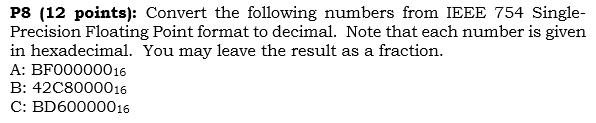P8 (12 points): Convert the following numbers from IEEE 754 Single- Precision Floating Point format to decimal. Note that each number is given in hexadecimal. You may leave the result as a fraction. A: BF00000016 B: 4208000016 C: BD60000016

• ### 2. Convert the following real numbers into single precision IEEE floating point format. Give the final...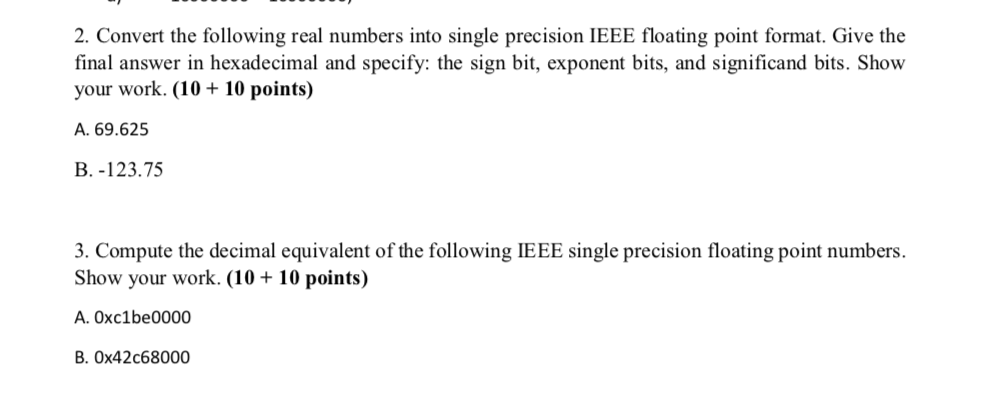2. Convert the following real numbers into single precision IEEE floating point format. Give the final answer in hexadecimal and specify: the sign bit, exponent bits, and significand bits. Show your work. (10 + 10 points) A. 69.625 B. -123.7 the following IEEE single precision floating point numbers. Show your work. (10 + 10 points) A. 0xc1be0000 B. 0x42c68000

• ### (2 pts) Express the base 10 numbers 16.75 in IEEE 754 single-precision floating point format. Express...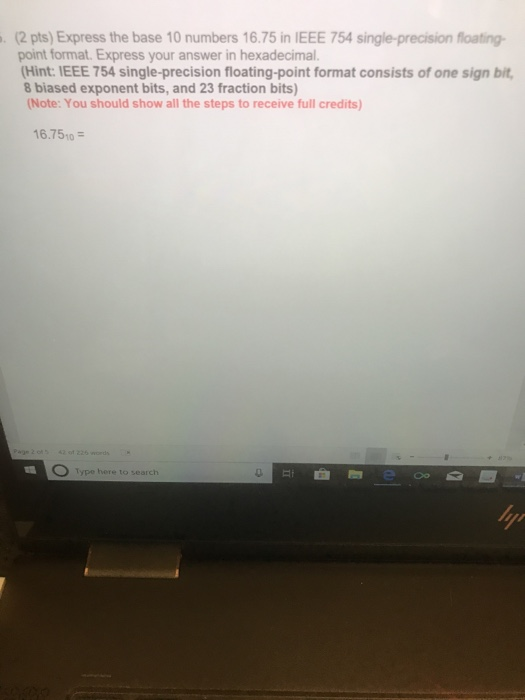(2 pts) Express the base 10 numbers 16.75 in IEEE 754 single-precision floating point format. Express your answer in hexadecimal. Hint: IEEE 754 single-precision floating-point format consists of one sign bit 8 biased exponent bits, and 23 fraction bits) Note:You should show all the steps to receive full credits) 6.7510 Type here to search

• ### 1 please IEEE-754 Floating point conversions problems (assume 32 bit machine): 1. For IEEE 754 single-precision...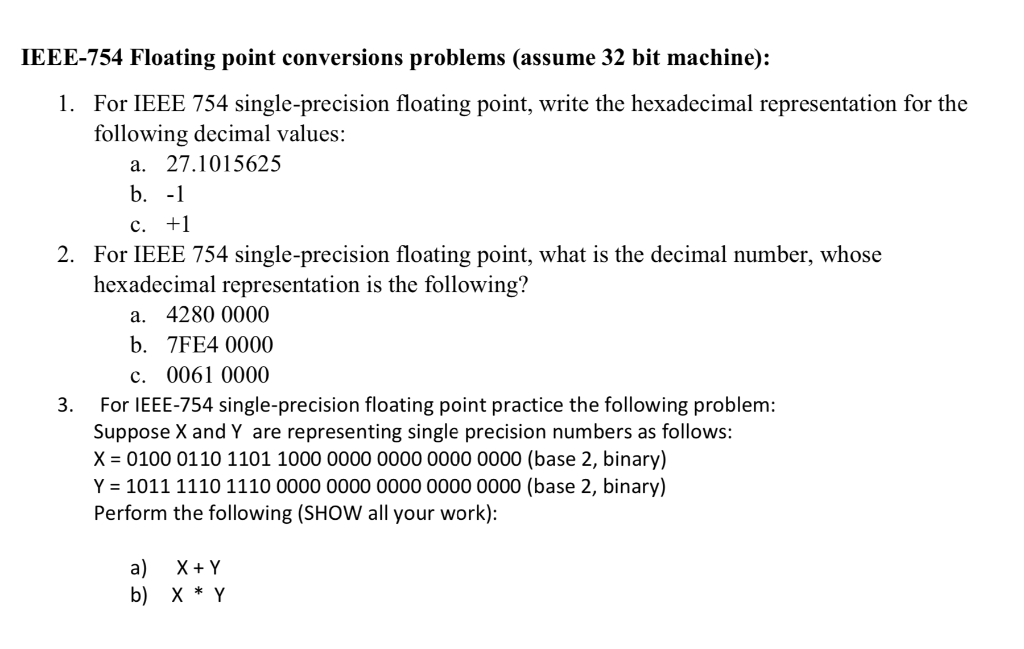1 please IEEE-754 Floating point conversions problems (assume 32 bit machine): 1. For IEEE 754 single-precision floating point, write the hexadecimal representation for the following decimal values: a. 27.1015625 b.-1 2. For IEEE 754 single-precision floating point, what is the decimal number, whose hexadecimal representation is the following? a. 4280 0000 b. 7FE4 0000 c. 0061 0000 3. For IEEE-754 single-precision floating point practice the following problem: Suppose X and Y are representing single precision numbers as follows: X 0100...

• ### This problem covers floating-point IEEE format. (a) Assuming single precision IEEE 754 format, what is the...

This problem covers floating-point IEEE format. (a) Assuming single precision IEEE 754 format, what is the binary pattern for decimal number -6.16? (b) Assuming single precision IEEE 754 format, what decimal number is represented by this word: 0 01111100 01100000000000000000000 (Hint: remember to use the biased form of the exponent.)

• ### For IEEE 754 single precision floating point, what is the number, as written in binary scientific...

For IEEE 754 single precision floating point, what is the number, as written in binary scientific notation, whose hexadecimal representation is the following? Show your work B350 0000 (hex)

• ### 5, [points] This problem covers floating-point IEEE format. (a) Assuming single precision IEEE 754 format, what...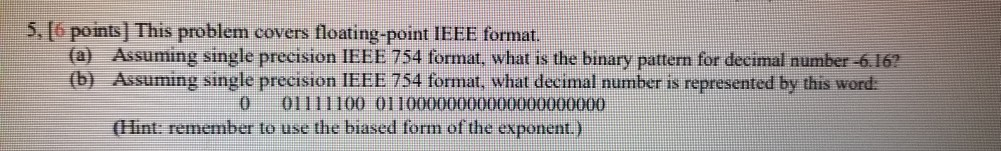5, [points] This problem covers floating-point IEEE format. (a) Assuming single precision IEEE 754 format, what is the binary pattern for decimal number -6.16? (b) Assuming single precision IEEE 754 format, what decimal number is represented by this word: 0 01111100 01100000000000000000000 (Hint: remember to use the biased form of the exponent.)

• ### 53. For IEEE 754 single precision floating point, what is the number, as written in binary...

53. For IEEE 754 single precision floating point, what is the number, as written in binary scientific notation, whose hexadecimal representation is the following? *(a) 4280 0000 (b) B350 0000 (c) 0061 0000 (d) FF80 0000 (e) 7FE4 0000 (f) 8000 0000

• ### 1. Convert the following decimal numbers in IEEE single-precision format. Give the result as eight hexadecimal...

1. Convert the following decimal numbers in IEEE single-precision format. Give the result as eight hexadecimal digits. a) -69/32 (-69 divide by 32) b) 13.625 2. Convert the following floating IEEE single-precision floating-point numbers from hex to decimal: a) 42E48000 b) C6F00040

• ### Please show steps EXERCICE4 The following real numbers are given in single precision (ieee-754 floating point)...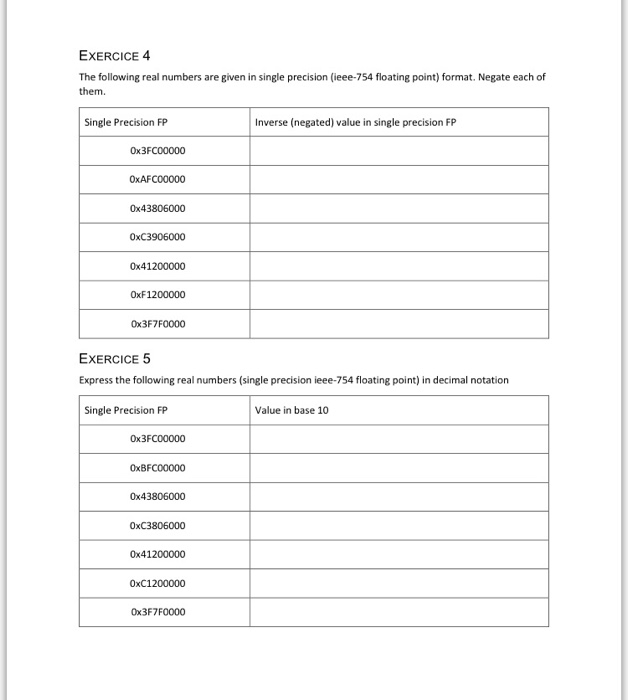Please show steps EXERCICE4 The following real numbers are given in single precision (ieee-754 floating point) format. Negate each of them. Single Precision FP Inverse (negated) value in single precision FP Ox3FCO0000 OxAFC00000 0x43806000 0xC3906000 0x41200000 0xF1200000 0x3F7F0000 EXERCICE 5 Express the following real numbers (single precision ieee-754 floating point) in decimal notation Single Precision FP Value in base 10 0x3FC00000 0xBFC00000 0x43806000 0xC3806000 0x41200000 0xC1200000 0x3F7F0000